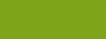﻿ Help 2Music Slide Rule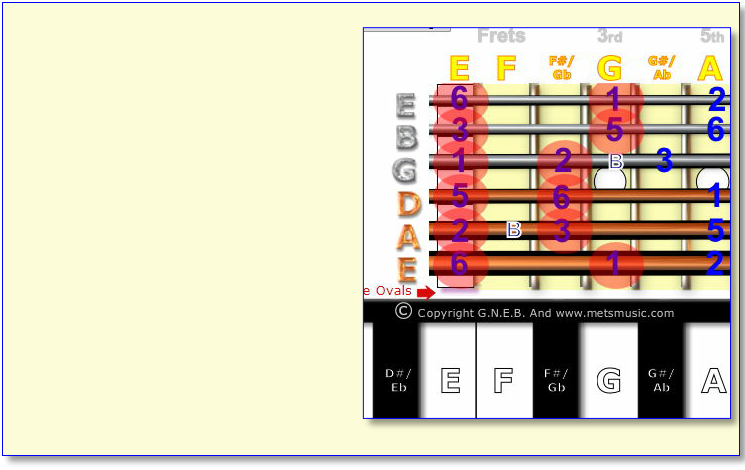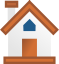HOME1st
Pentatonic and Blues Scales can be found two ways

1-The Hard Way
By using the Major Scales and Modes Slide Rule
and plotting out the formulas shown, or

2-The Easy Way
By using the Blues and Pentatonic Slide Rule
and following the numbers 1.2.B.3.5.6.

The 6th note of any Major Scale is known as the
relative minor e.g. in the Key of:
C Major. A minor is the relative minor.
C being the first note and A being the sixth note of
the C Major Scale

in the Key of:

G Major. E minor is the relative minor.
G being the first note and E being the sixth note of
the G Major Scale

So the Easy Way to find any Major Pentatonic Scale is
slide insert so the No. 1 is over the Key you want

Wherever the sixth note is will also be the
Minor Pentatonic.  See the Diagram 1 below.
Diagram 1  The Easy Way
using Pentatonic slide rule

The G Major Pentatonic Scale
here is the same as
the E minor Pentatonic Scale

No.1 is the Tonic G
(G)=G Major Pentatonic

No.6 is the Relative Minor E
(E)=E Minor Pentatonic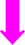Diagram 2  The Hard Way
using Major Scale slide rule

The Diagram here shows the
G Minor Pentatonic Scale
Plotted out using the Major Scale
Slide Rule

Notice this is the same as the
Bb Major Pentatonic Scale

But this can be a very confusing
Way to find the Pentatonic and or
Blues Scales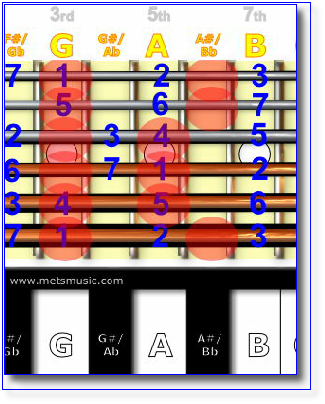2nd
In brief, the Easy Way is:
1-Use the Pentatonic Slide Rule

2-To find any Major Pentatonic Scale slide insert so
the No. 1 is over the Key you want

3-To find any Minor Pentatonic Scale slide insert so
the No. 6 is over the Key you want.  Easy
3rd
If you want to practice your soloing using the blues